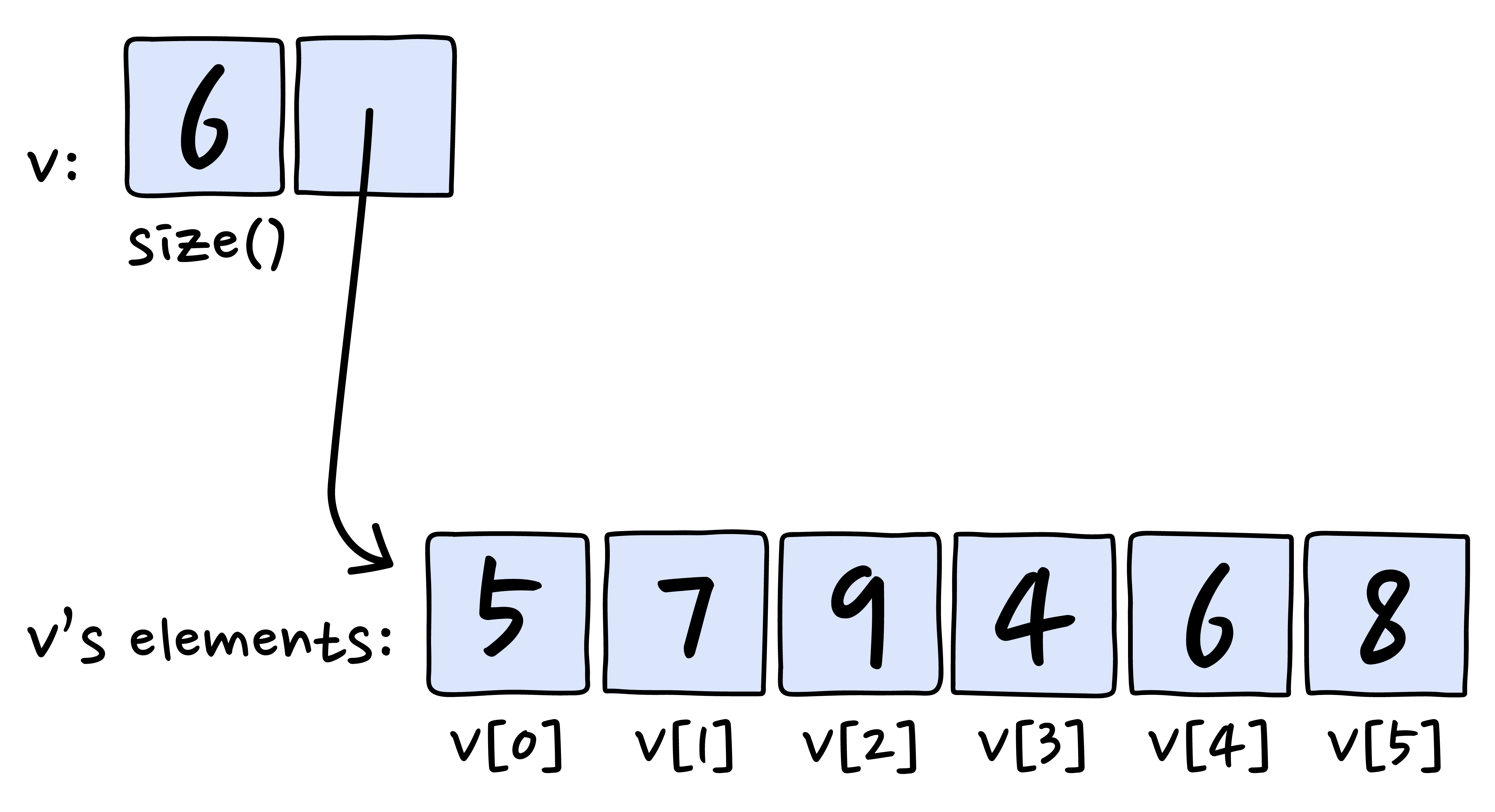Key Concepts

Review core concepts you need to learn to master this subject

C++ vectors

#include <iostream> #include <vector> using namespace std; int main() { vector<int> marks(2); // two int elements stored in variable marks marks = 100; marks = 200; cout << marks << endl; // displaying first element cout << marks; // displaying second element }

In C++, a vector is a dynamic array, which can shrink and grow in size. All the elements are stored in contiguous locations, so they can be accessed with an index. The index starts with the value 0.

To use vector we have to import vector library.

Adding and removing elements from vector

#include <iostream> #include <vector> using namespace std; int main() { vector<int> marks(2); // two int elements stored in variable marks marks = 100; marks = 200; cout << marks << endl; // displaying first element cout << marks; // displaying second element }

In C++, we use push_back() to add an element to a vector, and pop_back() to remove an element from vector.

index in C++.

#include <iostream> #include <vector> using namespace std; int main() { vector<int> marks(2); // two int elements stored in variable marks marks = 100; marks = 200; cout << marks << endl; // displaying first element cout << marks; // displaying second element }

In C++, to access a vector or an array element we use the index. The index starts from the value 0.

C++ Vector Type

#include <iostream> #include <vector> using namespace std; int main() { vector<int> marks(2); // two int elements stored in variable marks marks = 100; marks = 200; cout << marks << endl; // displaying first element cout << marks; // displaying second element }

During the creation of a C++ vector, the data type of its elements must be specified. Once the vector is created, the type cannot be changed.

size() function for a vector in C++

#include <iostream> #include <vector> using namespace std; int main() { vector<int> marks(2); // two int elements stored in variable marks marks = 100; marks = 200; cout << marks << endl; // displaying first element cout << marks; // displaying second element }

In C++, we use .size() function to get the number of elements in a vector.

Vectors
Lesson 1 of 1
1. 1
To do just about anything of interest in a program, we need a group of data to work with. For example, our program might need: - A list of Twitter’s trending tags - A set of payment options for a…
2. 2
The std::vector lives in the header. So first, we need to add this line of code at the top of the program: #include For review, #include is a preprocessor directive that tells the compiler t…
3. 3
Now we know how to create a vector, we can also initialize a vector, giving it values, as we are creating it in the same line. For example, instead of just creating a double vector named location:…
4. 4
Now that we have a vector, how do we access an individual element? This is where index comes into play. An index refers to an element’s position within an ordered list. Vectors are 0-indexed, me…
5. 5
Often, we start with a vector that’s either empty or a certain length. As we read or compute data we want, we can grow the vector as needed. ##### .push_back() ##### To add a new element to the “…
6. 6
not only stores the elements; it also stores the size of the vector:The .size() function returns the num…
7. 7
So what happens when you want to change each of the values within a vector? You can use a for loop! For example, suppose we have an int vector that looks like this: !(https://i.imgur.com/0MQd…
8. 8
Congratulations! You have learned about how to store groups of data into vectors in C++.  Here are some of the things that we learned: - Vectors are a sequence of elements that you can access by…

What you'll create

Portfolio projects that showcase your new skillsHow you'll master it

Stress-test your knowledge with quizzes that help commit syntax to memory